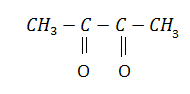${\mathrm{CH}}_{3}\mathrm{C}\equiv \mathrm{C}.{\mathrm{CH}}_{3}$In the above reaction X is:

(a) ${\mathrm{HNO}}_{3}$                                (b) ${\mathrm{O}}_{2}$

(c) ${\mathrm{O}}_{3}$                                     (d) ${\mathrm{KMnO}}_{4}$